# Binary Search Tree (BST)

Updated:

⚠️ This post was created when I was in high school and is no longer maintained.

## 1. Binary search recap

algorithm BinarySearch(A, key, start, end):
if start < end then
mid := floor((start + end) / 2)
if key == A[mid] then
return mid
if key < A[mid] then
BinarySearch(A, key, start, mid-1)
if key > A[mid] then
BinarySearch(A, key, mid+1, end)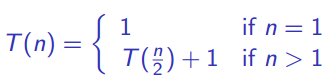=> Binary search takes Θ(log~2~n) time

## 2. Intuition

### 2.1 Components of a BST

- Two values: key and satellite data
- Three pointers: left, right, parent  ![ccf68e7ad840ffd79b66e50d142fa114.png](http://hongyu.nl/_resources//25d79aa449f64240b8843edce1b9400b.png)


### 2.2 BST property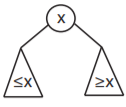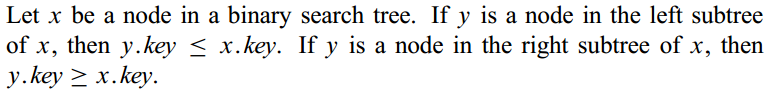Tips: Be careful that a BST is NOT an almost complete binary tree!!!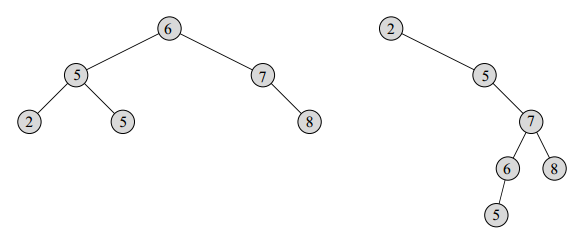## 3. BST operations

### 3.1 Searching

TREE-SEARCH: returns a pointer to a node with key k if one exists; otherwise, it returns NIL. The procedure begins its search at the root and traces a simple path downward in the tree. For each node x it encounters, it compares the key k with x.key

{- @x: starting node
- @k: target key
-}
-- recursive version --
algorithm TreeSearch(x, k)
if x == NIL or x.key == k then
return x
if k < x.key then
TreeSearch(x.left, k)
else
TreeSearch(x.right, k)

-- iterative version --
algorithm TreeSearch(x, k):
While x != NIL and x.key != k do
if k < x.key then
x = x.left
else
x = x.right
return x


Tip: in TreeSearch(), the sequence of nodes encountered forms a simple path downward from the root.

### 3.2 Minimum and Maximum:

We can always find an element in a binary search tree whose key is a minimum by following left child pointers from the root until we encounter a NIL —— CRLS

-- we assume that the input node is non-NIL --
algorithm TreeMinimum(x):
While x.left != NIL do
x = x.left
return x

algorithm TreeMaximum(x):
While x.right != NIL do
x = x.right
return x


### 3.3 Successor and Predecessor

The structure of a binary search tree allows us to determine the successor/predecessor of a node without ever comparing keys. ——　CRLS

• Successor:

If all keys are distinct, the successor of a node x is the node with the smallest key greater than x.key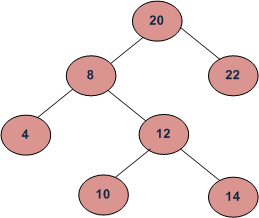In the above diagram, the inorder successor of 8 is 10, the inorder successor of 10 is 12 and inorder successor of 14 is 20.

• Predecessor:

If all keys are distinct, the predecessor of a node x is the node with the greatest key smaller than x.key

algorithm TreeSuccessor(x):
-- there are two cases --
{-  1. node x has the right subtree:
- find the smallest node in its right subtree
-}
if x.right != NIL then
return TreeMinimum(x.right)

{-  2. node x doesn't have right subtree:
- find the *lowest* ancestor of x whose left child
- is x.
-}
y := x.parent
While y != NIL and x == y.right do
--> (once x is no longer the right child of y, x > y)
x = y
y = y.parent

algorithm TreePredecessor(x):
-- the opposite --



Final notes:

• Recall that every node is its ancestor.
• The worst-case relies on the height of the tree (height matters).

### 3.4 Insertion

BST insertion is nothing but fnding a correct leaf place (NIL) for the newly inserted node:

{-  Assume keys are distinct and n is the new node that need inserting
- with z.key = v and z.left == z.right == NIL
-}
algorithm TreeInsert(T, n):
trailing := NIL --> y (in the book) is the trailing pointer that represnts the parent of x
target   := T.root
While target != NIL do --> Newly inserted node is always a leaf so we need to find a proper NIL for it
trailing := target
if n.key < target.key then
target = target.left
else
target = target.right
n.parent := traling --> this is where the trailing pointer comes into play (set the parent pointer of the new node)

if trailing == NIL then --> empty tree
T.root = n
else if n.key < trailing.key then
trailing.left = n
else
trailing.right = n


[Question]: why we need trailing pointer?

… This NIL occupies the position where we wish to place the input item n. We need the trailing pointer y because by the time we find the NIL where n belongs, the search has proceeded one step beyond the node that needs to be changed. —— CLRS

By saying “one step beyond”, it means we cannot use a NIL node to access its parent node that is needed to set n.parent (like a singlely linked-list). In other words, we use the trailing pointer to tack the parent when performing searching.

### 3.5 Deletion

There are 3 cases: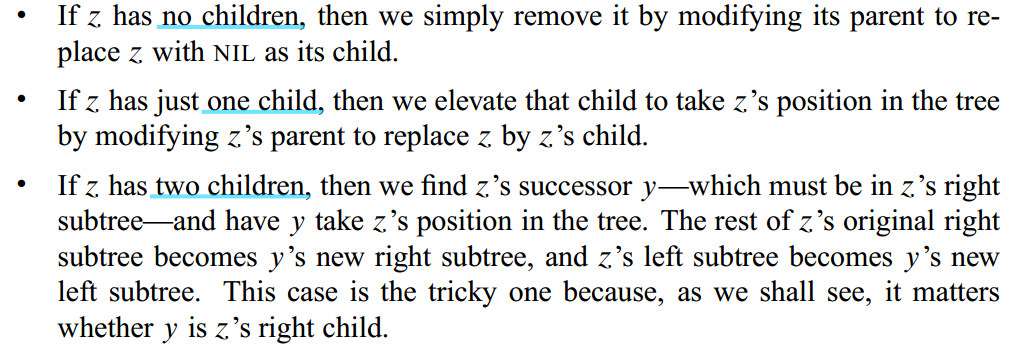Sum up: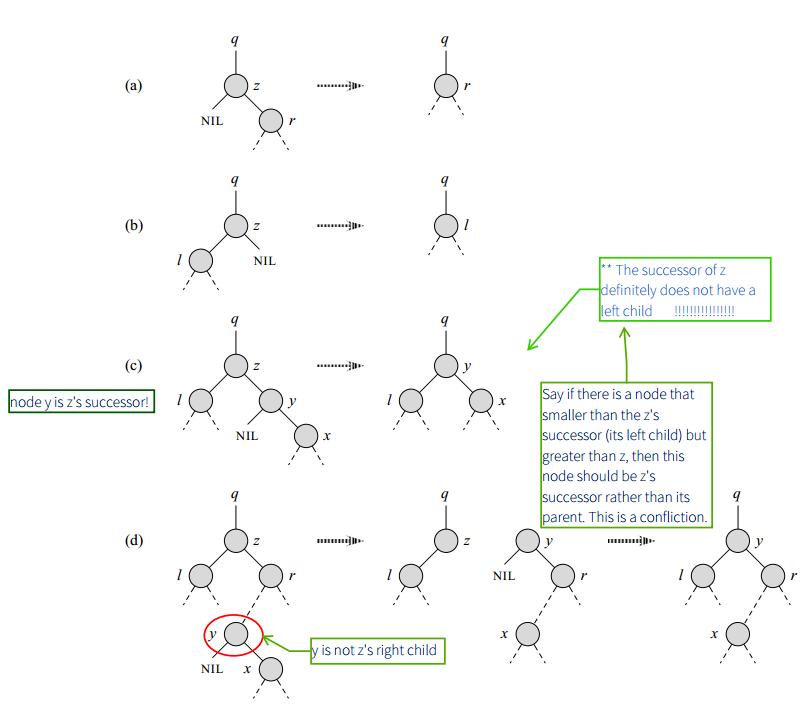(say z is the node that is wanted to be deleted) If z has:

• one child / single child: replace the deleted node by NIL or its child directly; -> situation: (a), (b)
• two children:
1. first, we need to find z’s successor node y which must be in the right subtree of z; (tips: y has no left child <- PBC see my book notes)
2. elevate y’s child to take y’s position
3. repace z by y and give y all z’s children

This eventually boils down to find the successor of the removed node and transplant it.

{-- TRANSPLANT replaces one subtree as a child of its
- parent with another subtree. When TRANSPLANT replaces
- the subtree rooted at node 'old' with the subtree
- rooted at node 'new', node 'old'’s parent becomes node
- 'new'’s parent, and 'old'’s parent ends up having
- 'new' as its appropriate child.
-}
algorithm Transplant(T, old, new):
-- 'old' is the root --
if old.parent == NIL then --> Why not old == T.root?
T.root = new
-- set parent node --
else if old == old.parent.left then
old.parent.left = new
else
old.parent.right = new
-- set parent pointer --
if new != NIL then
new.parent = old.parent

---------------------------------------

algorithm TreeDelete(T, z):
-- case (a), (b): single child --
if z.left == NIL then
Transplant(z, z.right)
else if z.right == NIL then
Transplant(z, z.left) --> replace directly (assume all subtrees satisfy BST property)
else
-- case (c), (d): two children --
successor := TreeMinimum(z.right) --> y
if successor.parent != z then
-- case d (prepare successor) --
Transplant(T, successor, successor.right)
-- update right child --
successor.right = z.right
successor.right.parent = successor

-- case c, d (replace z by its successor) --
Transplant(T, z, successor)
-- update left child --
successor.left = z.left
successor.left.parent = successor



Tips: the most time-consuming procedure lies in the TreeMinimum() which may take Θ(logn) time.

## 4. Time Complexity

1. All TreeSearch() TreeMinimum() TreeMaximum() TreeSuccessor() TreePredeseccor() take O(h) time on a binary search tree of height h.

2. For TreeDelete(), Tranplant() takes constant time only TreeMinimun() takes O(h) time, so both TreeInsert() and TreeDelete() take O(h) time on a binary search tree of height h.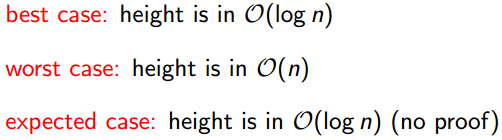## 5. Intermezzo: How many BT with n nodes exist?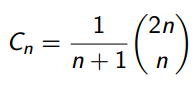first Catalan numbers: 1; 1; 2; 5; 14; 42; 132; 429; 1430; 4862; 16796; 58786; 208012; 742900; 2674440; 9694845; 35357670; 129644790; 477638700; 1767263190; 6564120420; 24466267020; 91482563640; 343059613650; 1289904147324; 4861946401452; …

Tags: